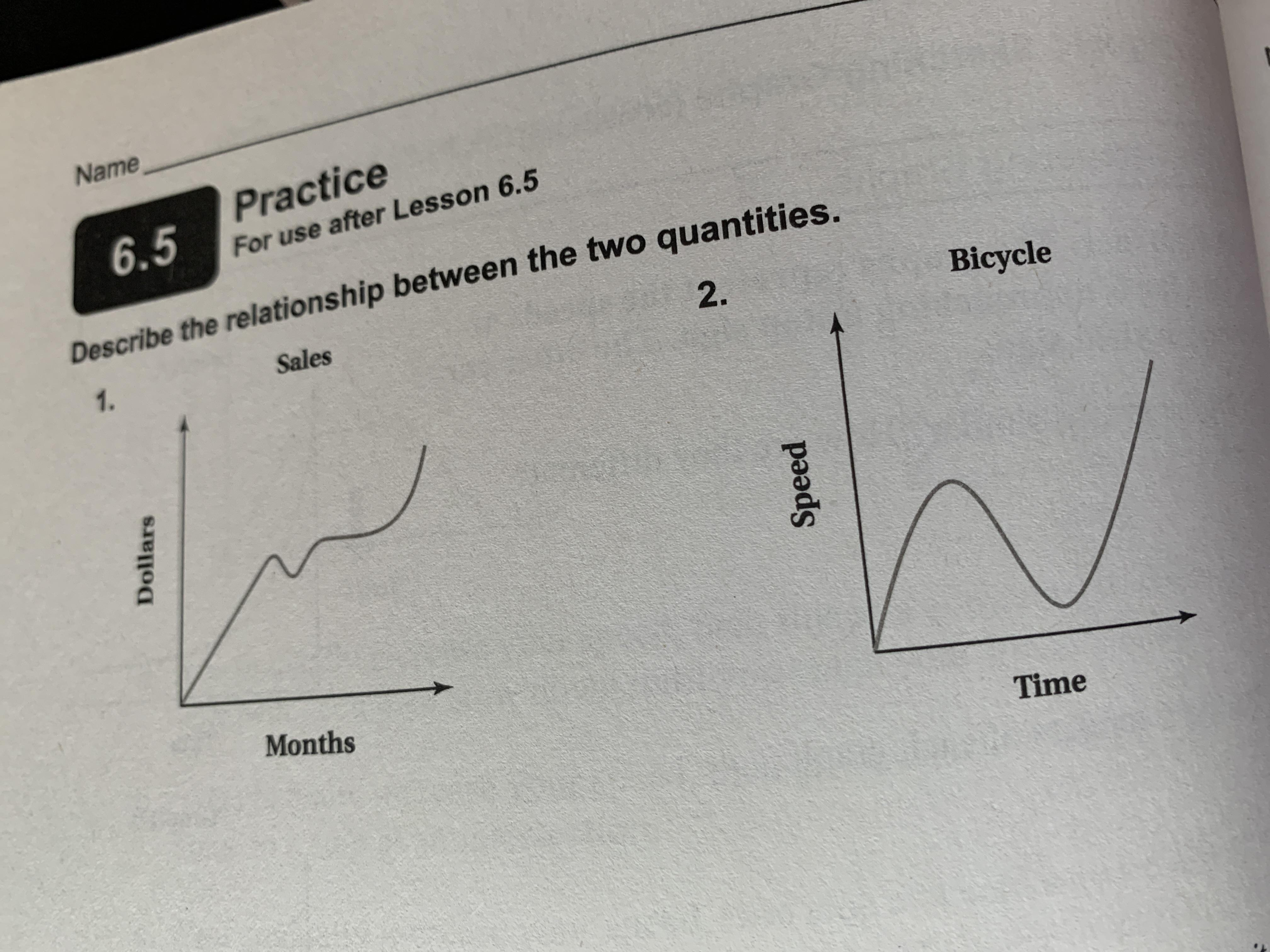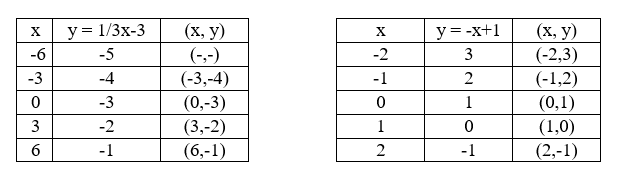Ask question

# What is the process to solve these:Vertex, y-int., x-int, graph= -(x+1)^2+1# What is the process to solve these:Vertex, y-int., x-int, graph= -(x+1)^2+1

Question
Linear equations and graphsasked 2021-02-09

What is the process to solve these:
Vertex, $$y-\int$$., $$x-\int$$, graph $$= -(x+1)^2+1$$

## Answers (1)2021-02-10

The intercepts are found by puttin $$gx=0 (y-\int)$$ and $$y=0 (x-\int)$$ into the parabola equation: $$\displaystyle{y}=-{\left({x}+{1}\right)}^{{2}}+{1}.$$
When $$x=0$$, $$y=-1+1=0$$, when $$y=0$$, $$\displaystyle{\left({x}+{1}\right)}^{{2}}={1}$$, so $$x+1=+-1$$, and $$x=-2$$ and 0.
That gives us 0 as y-int and -2 and 0 as x-ints. The graph is an inverted U shape where the arms of the U intersect the x-axis at -2 and 0, so the U lies to the left of the y axis.
The x value for the vertex is midway between the x intercepts at $$x=-1$$ and $$y=1 (-1,1)$$, and this can also be seen in the term $$(x+1)$$ as the displacement from the origin.

### Relevant Questionsasked 2020-12-03

A graph of a linear equation passes through ( -2,0) and (0,-6) is the $$3x-y=6$$, both ordered pairs solutions for the equationasked 2021-03-18

Describe the relationship between the two quantities.asked 2021-01-02

Solve linear equation and check: $$7x+3=6(x-1)+9$$asked 2021-01-31

Simplify each expression.
1) $$-n+9n+3-8-8n$$
2) $$3(-4x+5y)-3x(2+4)$$
3) $$5-4y+x+9y$$
4) $$-2x+3y-5x-(-8y)$$asked 2021-02-22

How to complete the table with the equation $$y=10x-4$$asked 2020-10-26

Determine the value of k for which the point (- 2, 3) lies on the line whose equation is .

$$4x+3ky=10$$asked 2021-02-19

$$y = -3x - 13$$ and $$4x + 3y = - 24$$asked 2020-12-13

A system of linear equations is a set of two or more equations taken together. The point where the two graphs intersect is called the solution.
$$\displaystyle{\left\lbrace\begin{array}{c} {y}=\frac{{1}}{{3}}{x}-{3}\\{y}=-{x}+{1}\end{array}\right.}$$
a) Complete the table fo each linear functionb) Graph both equations on the coordinate plane belowasked 2020-10-23

Determine whether the ordered pair is a solution to the given system of linear equations.
(1,2)
$$\left\{\begin{matrix} 3x−y=1 \\ 2x+3y=8 \end{matrix}\right\}$$asked 2020-10-23

Determine if (1,3) is a solution to the given system of linear equations.

$$5x+y=8$$

$$x+2y=5$$

...